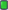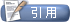MATLAB中国论坛|MATLAB爱好者之家—不仅仅是MATLAB![求助] 关于图像去噪的问题（附代码），求助大侠！！先谢谢了！
 用户名 记住信息 密码
 注册账号 论坛帮助 会员列表 日历事件 搜索 今日新帖 标记版面已读

 数字图像处理|SAR图像处理 A discussion board for Digital image processing & SAR image processing.2010-05-30, 10:32 PM #1 初级会员   注册日期: 2010-05-30 年龄: 32 帖子: 3 感谢他人: 0 有 0 帖获得 0 感谢 声望力: 0关于图像去噪的问题（附代码），求助大侠！！先谢谢了！ 各位大侠好： 我要实现基于直方图的高斯噪声滤波，我参考资料（关于去椒盐噪声的）实现了去除椒盐噪声的程序（加权中值法），那请问一下各位大侠，怎么才能用加权中值法去除高斯噪声？麻烦各位了，先谢谢了！ 附代码： I=imread('D:\Documents and Settings\Owner\实验2\2-原图.jpg'); figure(1); subplot(3,2,1),imshow(I);%显示原图像 subplot(3,2,2),imhist(I); J=imnoise(I,'salt & pepper',0.2);%加椒盐噪声 %J=imnoise(I,'gaussian',0.1);%加高斯噪声 subplot(3,2,3),imshow(J);%显示加椒盐噪声后的图像 subplot(3,2,4),imhist(J); [m,n,k]=size(J); newJ=J;% 随机生成一个矩阵newJ(m,n) dj=double(J); width_param=3;%窗口宽度参数 height_param=3;%窗口高度参数 window_width=2*width_param+1; window_height=2*height_param+1; %dj=uint8(dj); %figure(4); %subplot(2,2,1),imshow(dj);%显示加高斯噪声后的图像 %subplot(2,2,2),imhist(dj); h=zeros(1,256); for a=1:m %求和 for b=1:n h(J(a,b)+1)=h(J(a,b)+1)+1; end end w=zeros(1,256); for huidu=1:256 w(huidu)=h(huidu)/(m*n); end G=J; %初始化矩阵为1 for i=1:m for j=1:n G(i,j)=1; end end grayMatrix=G; for i=1:m for j=1:n max_value = 0;%最大灰度 min_value = 255;%最小灰度 i_min = i-width_param; if(i_min<1) i_min=1; end i_max = i+width_param; if(i_max>255) i_max=255; end j_min = j-height_param; if(j_min<1) j_min=1; end j_max = j+height_param; if(j_max >255) j_max =255; end for i1 =i_min:i_max; for j1 =j_min:j_max; if J(i1,j1)>max_value max_value=J(i1,j1); end if J(i1,j1)2010-05-31, 10:30 AM #2 初级会员   注册日期: 2010-05-30 年龄: 32 帖子: 3 感谢他人: 0 有 0 帖获得 0 感谢 声望力: 0回复: 关于图像去噪的问题（附代码），求助大侠！！先谢谢了！ 请大虾们帮看一下！！！！！怎样用加权均值滤波去除高斯噪声？？？？先谢谢了！！！！！！2010-05-31, 10:40 AM #3 初级会员   注册日期: 2010-05-30 年龄: 32 帖子: 3 感谢他人: 0 有 0 帖获得 0 感谢 声望力: 0回复: 关于图像去噪的问题（附代码），求助大侠！！先谢谢了！ 麻烦大侠帮看一下！！！！！！！！！先谢谢了！！！！！！主题工具显示可打印版本邮寄本页给好友 显示模式平板模式切换到混合模式切换到树形模式发帖规则 您不可以发表新主题 您可以发表回复 您不可以上传附件 您不可以编辑自己的帖子 启用 BB 代码 论坛启用 表情符号 论坛启用 [IMG] 代码 论坛禁用 HTML 代码Trackbacks are 禁用 Pingbacks are 禁用 Refbacks are 启用 版面规则

 -- 简体中文 -- 繁體中文 -- English (US) 联系我们 - MATLAB中国论坛|MATLAB爱好者之家 - 论坛存档 - 返回顶端